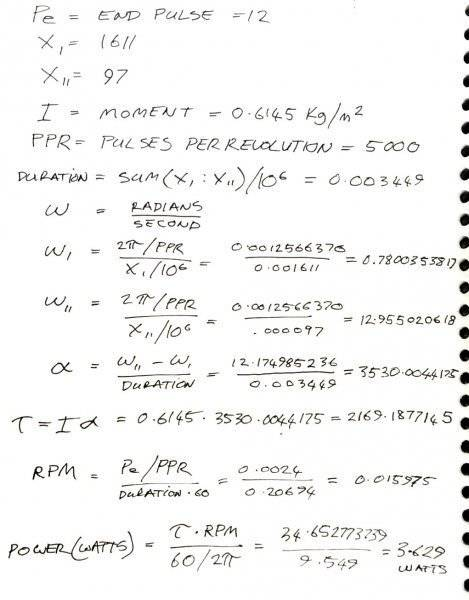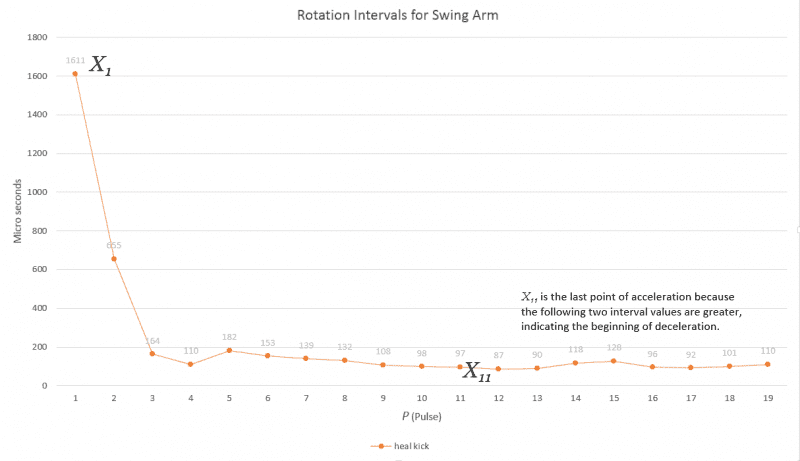# Finding power figures from graph data

I need help! Could you please double checking my work: calculating a Power information from data I am collecting. I have been Googling and wiki'ing for weeks now and I think I have it right but I need someone to confirm or correct what i have done..

The graph data comes from and Optical Rotary Encoder, which for the unfamiliar, is used to measure/feedback the position of a shaft. In my case I am using it to measure the acceleration of a shaft spin for this project. I have been working on the project for 2 years now and getting past this math stage would be a huge step towards completion.

also, sorry about the Sudo-notation . :)## Answers and Replies

mfb
Mentor
The formula you use for power assumes a constant rpm, something that is certainly not true.

During the first interval (lasting 1.6ms) the wheel accelerates a lot, assuming a uniform velocity within that timescale (to get an angular velocity) is not a good approximation. This does matter if you want to calculate the acceleration - but that gives larger errors anyway.
I would calculate the initial energy and the final energy, divide the difference by the time and you get the average power in a very model-independent way.

I would calculate the initial energy and the final energy, divide the difference by the time and you get the average power in a very model-independent way.

I will give this (above quoted) a go for sure.

In you reply you say that the 1.6 micro-second interval " assuming a uniform velocity within that timescale is not a good approximation." are you saying I am working with "bad" data? If so does this mean that i try your solution, or any solution, that will will yield inaccurate results?

mfb
Mentor
Well no method will give exact results as your data is available in those time steps only.
In particular, you do not know when exactly acceleration started, which gives an uncertainty of ~800µs.

Well no method will give exact results as your data is available in those time steps only.
In particular, you do not know when exactly acceleration started, which gives an uncertainty of ~800µs.

Yes I should have mentioned this earlier, the acceleration starts in the interval before X1. I didnt include it because it is impossible to know its real angle and is therefore null data. Does this effect what you have replied with so far?

mfb
Mentor
Then we don't know when the acceleration starts? Well, it increases the uncertainty.
If there is some physical motivation to assume a constant torque or a constant power, a fit to the data might give better results, but I don't think it helps here.

I would calculate the initial energy and the final energy, divide the difference by the time and you get the average power

I was wondering... do you think it would be more accurate to find the power for each of the 12 intervals and get the average from across those?

mfb
Mentor
That will give exactly the same result. But it might be interesting to remove the first interval. On the other hand, I would not expect power to be constant, so you have to figure out what exactly you want to calculate anyway.